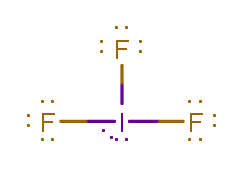# Problem: Predict the molecular geometries of the following species, respectively: IF3, TeF4, XeF5+. A. trigonal pyramidal, square planar, linearB. trigonal pyramidal, tetrahedral, see-saw C. trigonal planar, tetrahedral, square pyramidalD. T-shaped, see-saw, square pyramidal E. trigonal planar, see-saw, square planar

###### FREE Expert Solution

IF3

I     Group 7A   7 val. e- x 1 = 7 val. e-
F    Group 7A   7 val. e- x 3 = 21 val. e-
Total = 28 val. e-

I → less electronegative than F → central atom

Lewis structure:2 lone pairs and 3 atoms around central atom → AX3E2T-shaped

TeF4

Te  Group 6A   6 val. e- x 1 = 6 val. e-
F    Group 7A   7 val. e- x 4 = 28 val. e-
Total = 34 val. e-

Te → less electronegative than F → central atom

85% (409 ratings)###### Problem Details

Predict the molecular geometries of the following species, respectively: IF3, TeF4, XeF5+

A. trigonal pyramidal, square planar, linear

B. trigonal pyramidal, tetrahedral, see-saw

C. trigonal planar, tetrahedral, square pyramidal

D. T-shaped, see-saw, square pyramidal

E. trigonal planar, see-saw, square planar